# R语言旅行推销员问题TSP

## 原文链接：http://tecdat.cn/?p=6551

``````

coords.df <- data.frame(long=TSP225\$Long, lat=TSP225\$Lat)

coords.mx <- as.matrix(coords.df)

# Compute distance matrix

dist.mx <- dist(coords.mx)

# Construct a TSP object

tsp.ins <- tsp_instance(coords.mx, dist.mx )

#

tour <- run_solver(tsp.ins, method="2-opt")

#Plot

autoplot(tsp.ins, tour)``````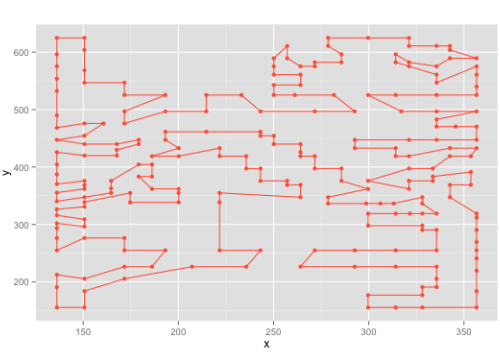?

``````methods <- c("nearest_insertion" "2-opt")

tours <- sapply(methods  simplify = FALSE)

dotchart( ),

)``````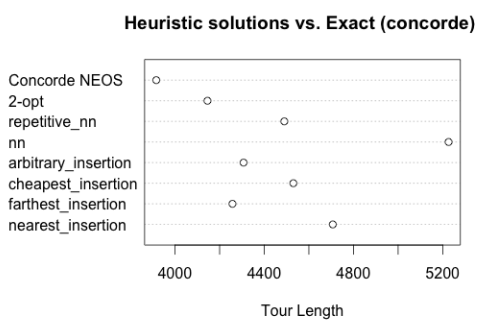?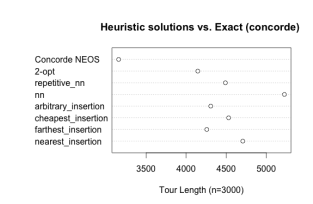?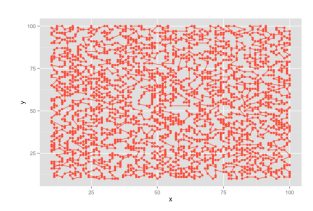?

## 非常感谢您阅读本文，有任何问题请在下面留言！?

【服务场景】

【大数据部落】提供定制化的一站式数据挖掘和统计分析咨询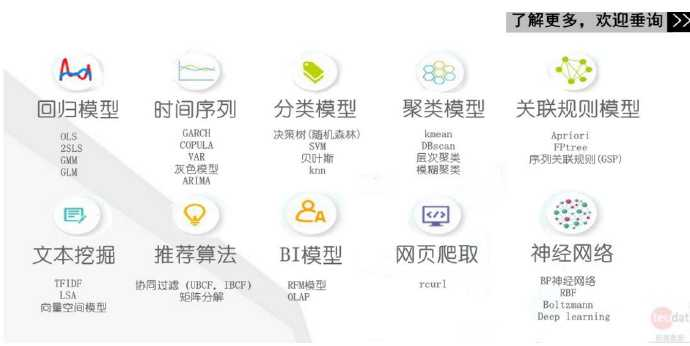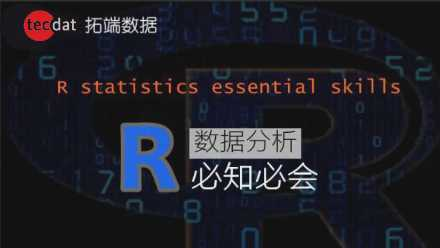R语言旅行推销员问题TSP

(0)
(0)

© 2014 bubuko.com 版权所有 鲁ICP备09046678号-4